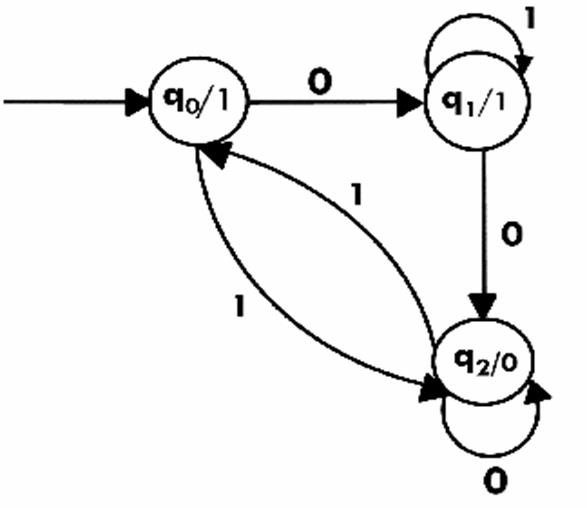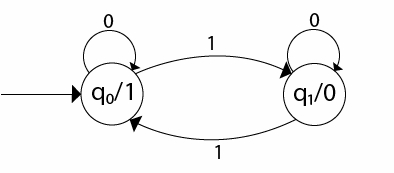# What is a Moore Machine in TOC?

Moore machine is a finite state machine in which the next state is decided by the current state and the current input symbol.

The output symbol at a given time depends only on the present state of the machine.

The Moore machine has 6 tuples

(Q, q0, Σ, O, δ, λ)

Where,

• Q: Finite set of states
• q0: Initial state of machine
• Σ: Finite set of input symbols
• O: Output alphabet
• δ: Transition function where Q × Σ → Q
• λ: Output function where Q → O

The state diagram is as follows −## Example 1

Input − 010

Transition − δ (q0,0) => δ(q1,1) => δ(q1,0) => q2

Output − 1110(1 for q0, 1 for q1, again 1 for q1, 0 for q2)

The transition table for Moore machine is as follows −

Current StateNext StateOutput
01
q0q1q21
q1q2q11
q2q2q00

## Explanation

• Step 1 − current state q0 on input ‘0’ it goes to state q1 and on ‘1’ goes to q2 generating output 1.
• Step 2 − q1 on input ‘0’ goes to state q2 and on ‘1’ goes to q1 generating output ‘1’.
• Step 3 − q2 on input ‘0’ goes to q2 and on ‘1’ goes to q0 generating output ‘0’.

## Example 2

Design a Moore machine whether an input string contains an even or odd number of 1's.

If input is even number of 1’s, the output is 1

Otherwise, the output is 0

The transition diagram is as follows −## Transition Table

The transition table is as follows −

Current stateNext state input 0Next state input 1Output
->q0q0q11
q1q1q00